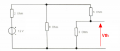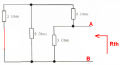#### waz

Joined Nov 14, 2014
16
Hi Guys, This is my first time on the forum so I am unsure whether I will get any replies on this post.

I am struggling on to do thevenins and nortons theory.

My question is attached.

Please help#### Jony130

Joined Feb 17, 2009
5,445
In which part you have troubles? Please show us your attempt. Because this is your homework not ours.

#### waz

Joined Nov 14, 2014
16
In which part you have troubles? Please show us your attempt. Because this is your homework not ours.

The first part of this question i have not shown, but i have already worked out the current using circuit reduction methods and found that the current passing through the 5 Ohm resistor is 0.38Amps.

Even though I already know what the current is I have to use both thevenins and Nortons merely to check my answer is correct.

I have no idea where to even start with either of them thats the problem.

Reading up on both the Thevenins and Nortons has confused me massively as it involves removing various parts of the circuit etc...? :/

#### Jony130

Joined Feb 17, 2009
5,445
We treat 5 ohm resistor as a load so we remove this resistor from the circuit.
And we left with thisAll you need to do now is to solve for Vth voltage.

After you find Vth we need to find Rth for this circuit (I replace voltage source with a short circuit ).Please find equivalent resistance seen by looking into A-B terminals.

Last edited:

#### waz

Joined Nov 14, 2014
16
We treat 5 ohm resistor as a load so we remove this resistor from the circuit.
And we left with this
View attachment 75612
All you need to do now is to solve for Vth voltage.

After you find Vth we need to find Rth for this circuit (I replace voltage source with a short circuit ).

View attachment 75613

Please find equivalent resistance seen by looking into A-B terminals.
This is the point that i get confused.

To work out the Vth do i have to use circuit reduction and work from there?

#### dalam

Joined Aug 9, 2014
58
Yes you have to do circuit reduction in order to get both Rth and Vth.
I guess Rth=2.15Ohm and Vth=2.71.
And Rth remains the same in both cases.
Find out Isc on your own.

#### Jony130

Joined Feb 17, 2009
5,445
Thevenin don't care about the method you use to find a solution for Vth. You can use any method you what/you know.

#### waz

Joined Nov 14, 2014
16
Thevenin don't care about the method you use to find a solution for Vth. You can use any method you what/you know.
I have no idea if any of this is correct.

Apologies for the messy writing i am doing it in rough for now.

does this make sense to anyone??

And am i doing the right things??

#### Jony130

Joined Feb 17, 2009
5,445
Why I2 is splits into I3 and I4 ? How can it be?
Also check your math. How can 1.92 * 0.54 equals 2.45??

#### waz

Joined Nov 14, 2014
16
Why I2 is splits into I3 and I4 ? How can it be?
Also check your math. How can 1.92 * 0.54 equals 2.45??

Sorry i was rushing and put the wrong number in the calculator.

Is my method correct up to where i made this mistake?

I really have no idea what to do on this, I am reading online how to find the Vth but nothing makes sense to me. Likewise with Rth?

#### Jony130

Joined Feb 17, 2009
5,445
Once i have the I1 and I2 currents what do i do next?
Simply use the Ohm's law and find Vth voltage.

#### waz

Joined Nov 14, 2014
16
Simply use the Ohm's law and find Vth voltage.
If i start from the beginning again
,Am i correct to do:
6+3=9 ohms as resistors in series are added.

I can then do product/sum= 8 x 9/ 8+9= 4.24 ohms

2 ohm + 4.24 ohms= 6.24 ohms.

So the circuit will contain the supply and one resistor.

is this correct so far?

#### Jony130

Joined Feb 17, 2009
5,445

#### waz

Joined Nov 14, 2014
16
Everything is ok so far
Then do:

It= V/Rt= 12/6.24 =1.92 amps

Now i can calculate the current on the circuit putting back the other resistors. So i have a 12v supply, 2 ohm, 8 ohm and 9 ohm resistor.

I1= It x 9/17
I1= 1.92 x 0.53
I1= 2.45 amps

I2= It x 8/17
I2= 1.92 x 0.47
I2= 2.39 amps

Is this ok so far?

#### Jony130

Joined Feb 17, 2009
5,445
I1= It x 9/17
I1= 1.92 x 0.53 = 2.45 amps

I2= It x 8/17
I2= 1.92 x 0.47 = 2.39 amps
Some kind of a new math? Check your math or use a google to do the math for you.

#### waz

Joined Nov 14, 2014
16
Some kind of a new math? Check your math or use a google to do the math for you.
Apologies.

I1= It x 9/17
I1= 1.92 x 0.53 = 1.01 amps

I2= It x 8/17
I2= 1.92 x 0.47 = 0.90 amps

Where do i go next?

#### Jony130

Joined Feb 17, 2009
5,445
Vth is a voltage across 3 ohm's resistor, so all you need is to use Ohm's law and find Vth.